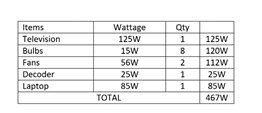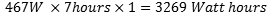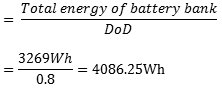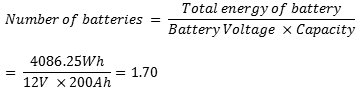# What size of battery bank do I need in my home?

How to calculate the size of the battery bank needed for your home?

We know that batteries are referred to as the life of an inverter system. How well your inverter serves you is dependent on the quality of your battery, design and more.

Previously, we discussed how to calculate the size of inverter needed for your home. Based on that, We came to a conclusion that based on that load, you need a 0.8kVA inverter. Batteries used for homes usually have capacities ranging from 75Ah to over 300Ah.Recall that the total power requirement was 467 Watts. Let’s assume you want to run the system for 7 hours when there’s a power outage. Let’s calculate the number of batteries you’ll need in the next few steps:

Assume:

Back-up time: 7 hours

Days of autonomy: 1
Days of autonomy means the number of days a battery bank can provide the appliances you have connected to the system without being recharged.

### Calculate the total energy of the battery bank

Total Energy of the battery bank = (Total Load (W) x Backup Hours) x Days of Autonomy;### Divide by the Depth of Discharge of the battery.

ALSO READ: What is Depth of Discharge?

Let’s assume your DoD is 80%.### Total number of batteries

Divide the total energy of the battery (Assuming you’re using a 200Ah battery for your design. It could 150AH) by the Battery Voltage x Capacity.Approximately, that will be 2 batteries. Therefore, you will need two, 12V 200Ah batteries to power your load for 7 hours.

Did you know we have a weekly tweet-chat – #LearnWithHHS on where we discuss questions popularly asked and profer solutions to problems in the renewable energy (solar) industry? Join us every Wednesday at 3:30 PM WAT.